I apologize. It has been too long. I took a long break from blogging because I was busy and burnt out. Without getting into too much detail as to why I felt burnt out, I shall briefly state that working with a couple incompetent partners back to back is enough to burn anybody out. After witnessing all the drama, the greed, the deception, and even the swindling of company funds, I have had enough. I would rather jump back into a 9 to 5 and earn a steady, comfortable paycheck.

…which is exactly what I did. I even worked briefly at a large .NET shop staying under the radar and coding quietly in C#. That’s how bad it was; I worked at a Microsoft shop.

I tried everything to recover from this burnout, short of changing careers.

During these times, one major activity I picked up to assist in my recovery and escape from my stresses was sporting clays. For those that aren’t familiar with sporting clays, it is a challenging (but fun and addicting) shotgun shooting sport that began as simulated hunting. Unlike skeet and trap, sporting clays requires the shooter to migrate from station to station (usually 10 or more stations) either on foot or golf cart. At each station there are a pair of clay throwers that throw clay targets in a wide variety of presentations. No two stations are alike, and the shooter must shoot each pair as either a true pair (two targets at once) or a report pair (one target first, second target immediately after the first shot). The targets can fly overhead, come towards you, drop, cross, roll, etc. Scores are kept and represented as number of total targets broken. The easiest way to describe this sport is “golf with a shotgun”. It’s no wonder sporting clays is currently the fastest growing shooting sport.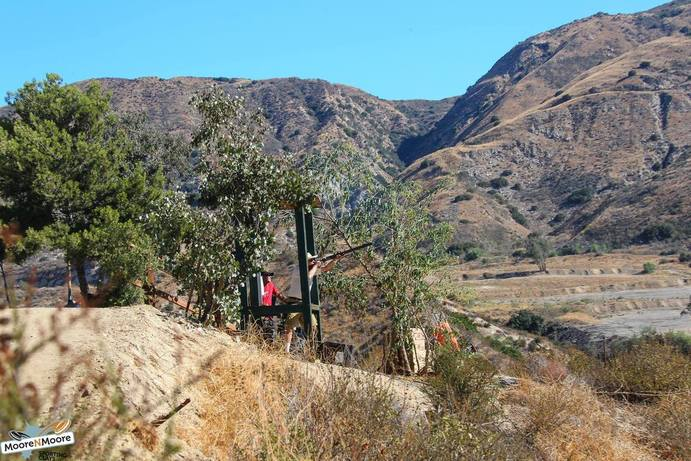You’re probably wondering why I’m talking about shooting. Well, as I became more involved in the sport, I began to analyze the targets in order to improve my score. It turns out to be a very fun problem to solve which involves a bit of trigonometry, physics, and software engineering.

Let’s begin with the knowns. A shooter is typically firing 1 oz or 1 1/8 oz of lead (7 1/2 or 8 shot) downrange at anywhere from 1100 to 1300 feet per second. The clay targets are typically thrown at 41 mph but can vary. Rarely, targets can be launched at blazing speeds up to 80 mph. The direction and position of the clay throwers are always different, but shots are usually expected to be taken in the 20-50 yard range. On occassion, you may be expected to take an 80 yard shot (or further) but that would be extremely rare. The “breakpoint” is where the shot meets the target and breaks the target.

Since we’re not shooting laser rifles, there’s a certain amount of “lead” seen by the shooter or else he/she would be missing from behind. So how do we calculate this lead?
I consider there to always be two different types of leads: the actual lead (how far ahead the pattern actually needs to be) and the visual lead (how the lead appears to the shooter from the shooter’s perspective)

For example, if a target was a straightaway target, all we would have to do is shoot right at it, making the “actual lead” unimportant and the “visual lead” non-existent. If a target was a 90 degree crosser, perfectly perpendicular to the gun’s shot path, that would simply require a conversion of miles per hour to feet per second (5280 feet = 1 mile) and determining how much quicker the shot pattern reaches the breakpoint before the clay target. But of course, nothing is this simple. The truth is, breakpoints vary, angles vary, distances vary, velocities vary, thus leads vary. Even the same target thrown from the same machine will have a different lead depending on where in its flight path you decide to shoot it.

This is how I began to tackle the problem:1) I visualize the different points. S = shooter, T = thrower, B = breakpoint, P = target location.2) I determine the distance between shooter and the breakpoint.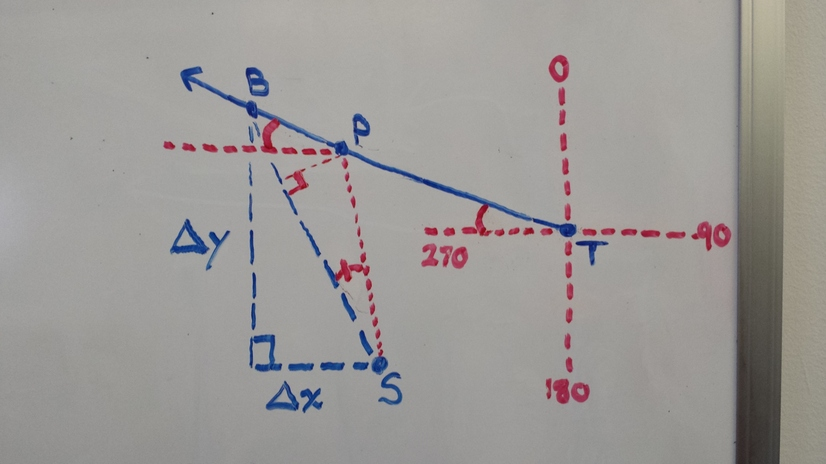3) I determine the shooter’s angle between the breakpoint and the target location… in other words, the lead in degree angles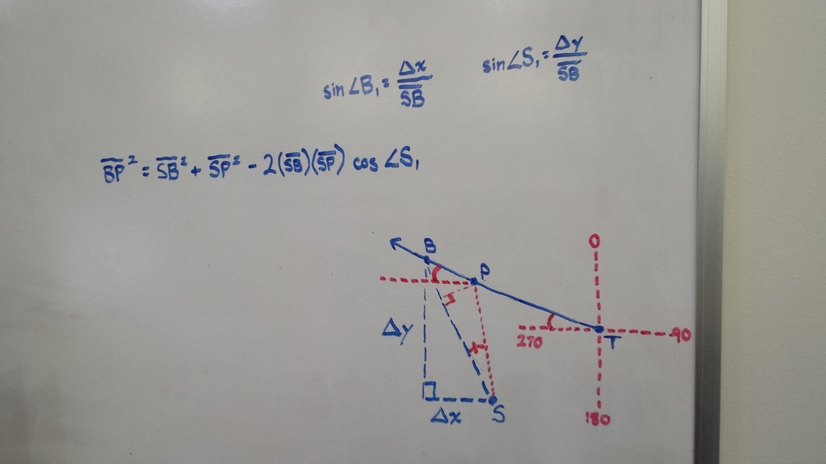4) I determine the distance (actual lead).5) I determine the visual lead which is actually just an adjacent side to the right angle of the triangle and opposite to the lead in degree angles.
6) I code this up using Python

Here is my implementation:

from __future__ import division
import math

class UnitConversionsMixin(object):
'''
Unit conversion calculations
5280 feet = 1 mile
'''

@classmethod
def fps_to_mph(cls, fps):
'''
converts fps to mph
'''
return (fps / 5280) * 3600

@classmethod
def mph_to_fps(cls, mph):
'''
converts mph to fps
'''
return (mph * 5280) / 3600

@classmethod
def angle_to_thumbs(cls, angle):
'''
converts degree angle to thumbs.
this method assumes the average human thumb width is approximately 2 degrees
'''
return angle / 2

class TrigonometryMixin(object):
'''
Trigonometric calculations
'''

@classmethod
def angle_by_sides(cls, a, b, c):
# applies law of cosines where we are trying to return the angle C (opposite corner of c)
cos_C = (c**2 - b**2 - a**2) / (-2 * a * b)
C = math.acos(cos_C)
return math.degrees(C)

@classmethod
def side_by_angles_and_side(cls, a, angle_a, angle_b):
# applies law of sines where we are trying to return the side b (opposit corner of angle B)
return b

class Shooter(object):
'''
Represents a shooter
'''
velocity = 1200  # velocity of shotshell in feet per second
position = (0,0)  # position of shooter in cartesian coordinates (x,y).  This should always be (0,0)
direction = 0  # direction in which the station is pointing in degree angle 0 = 360 = 12 o'clock. 90 = 3 o'clock. 180 = 6 o'clock. 270 = 9 o'clock.

def __init__(self, velocity=1200, direction=0):
self.velocity = velocity

class Thrower(object):
'''
Represents a thrower
'''
position = (0,0)  # position of thrower in cartesian coordinates (x,y) where each unit of measurement is in feet
velocity = 41  # velocity of clay targets in miles per hour
direction = 0  # direction of clay target trajectory in degree angle 0 = 360 = 12 o'clock. 90 = 3 o'clock. 180 = 6 o'clock. 270 = 9 o'clock.
destination = (40,40) # position of destination of target in cartesian coordinates (x,y) where each unit of measuremnt is in feet

def __init__(self, position, direction=None, destination=None, velocity=41):
self.position = position
self.direction = direction
self.velocity = velocity
self.destination = destination
if not self.velocity and not self.destination:
raise Exception('You must specify either a direction (angle) or destination (end position)')
if direction is None:
self.direction = self.destination_to_direction(destination)

def direction_to_destination(self, direction, distance=100, offset=None):
#import ipdb; ipdb.set_trace()
hypotenuse = distance
if offset is None:
offset = self.position
if direction &gt; 270:
angle = 360 - direction
x_diff = math.sin(rads) * hypotenuse * -1
elif direction &gt; 180:
angle = direction - 180
y_diff = math.cos(rads) * hypotenuse * -1
x_diff = math.sin(rads) * hypotenuse * -1
elif direction &gt; 90:
angle = 180 - direction
y_diff = math.cos(rads) * hypotenuse * -1
else:
angle = direction
return (round(x_diff + offset, 2), round(y_diff + offset, 2))

def destination_to_direction(self, destination):
x_diff = destination - self.position
y_diff = destination - self.position
hypotenuse = math.sqrt(x_diff**2 + y_diff**2)
cos_angle = abs(y_diff) / hypotenuse
angle = math.degrees(math.acos(cos_angle))
if x_diff &gt;= 0:
if y_diff &gt;= 0:
direction = angle
else:
direction = 180 - angle
else:
if y_diff &gt;= 0:
direction = 360 - angle
else:
direction = 180 + angle
return direction

'''
'''

@classmethod
def _get_angle_by_sides(cls, a, b, c):
# applies law of cosines where e are trying to return the angle C (opposite of side c
cos_C = (c**2 - b**2 - a**2) / (-2 * a * b)
C = math.acos(cos_C)
return math.degrees(C)

@classmethod
# breakpoint location in cartesian coordinates tuple(x,y)

# find breakpoint distance from shooter
shot_x_diff = breakpoint - shooter.position
shot_y_diff = breakpoint - shooter.position
shot_distance = math.sqrt(shot_x_diff**2 + shot_y_diff**2)
shot_time = shot_distance / shooter.velocity
target_diff = cls.mph_to_fps(thrower.velocity) * shot_time

# reverse direction
reverse_direction = (thrower.direction + 180) % 360
target_location = thrower.direction_to_destination(reverse_direction, target_diff, breakpoint)

# find target distance from shooter at moment of trigger pull
pull_x_diff = target_location - shooter.position
pull_y_diff = target_location - shooter.position
target_distance = math.sqrt(pull_x_diff**2 + pull_y_diff**2)

# find lead in thumb widths

# find visual lead in ft

return {
'breakpoint': breakpoint,
'pullpoint': target_location,
'shot_distance': round(shot_distance, 2),
'target_distance': round(target_distance, 2),
'trajectory': round(thrower.direction, 2)
}

@classmethod
# shooter angle in degrees 0 = 360 = 12 o'clock. 90 = 3 o'clock. 180 = 6 o'clock. 270 = 9 o'clock

# find distance from shooter to thrower
delta_x = thrower.position - shooter.position
delta_y = thrower.position - shooter.position
thrower_shooter_distance = math.sqrt(delta_x**2 + delta_y**2)

# find angle to thrower
cos_angle = abs(delta_y) / thrower_shooter_distance
angle_to_thrower = math.degrees(math.acos(cos_angle))
if delta_x &gt;= 0:
if delta_y &gt;= 0:
pass
else:
angle_to_thrower = 180 - angle_to_thrower
else:
if delta_y &gt;= 0:
angle_to_thrower = 360 - angle_to_thrower
else:
angle_to_thrower = 180 + angle_to_thrower

thrower_to_shooter_angle = (angle_to_thrower + 180) % 360

# get breakpoint distance from shooter

# get breakpoint distance from thrower

# get breakpoint location
breakpoint = thrower.direction_to_destination(thrower.direction, breakpoint_distance_from_thrower)

# get shot time
shot_time = shot_distance / shooter.velocity

target_diff = cls.mph_to_fps(thrower.velocity) * shot_time

# reverse direction
reverse_direction = (thrower.direction + 180) % 360
target_location = thrower.direction_to_destination(reverse_direction, target_diff, breakpoint)

# find target distance from shooter at moment of trigger pull
pull_x_diff = target_location - shooter.position
pull_y_diff = target_location - shooter.position
target_distance = math.sqrt(pull_x_diff**2 + pull_y_diff**2)

# find lead in thumb widths

# find visual lead in ft

return {
'breakpoint': breakpoint,
'pullpoint': target_location,
'shot_distance': round(shot_distance, 2),
'target_distance': round(target_distance, 2),
'trajectory': round(thrower.direction, 2)
}


Of course since not all the triangles represented in this diagram are right triangles, I would have to utilize the law of cosines and law of sines to find certain distances as well as the angles.

Using my software, I conducted tests with the shooter shooting 1200 fps shot at a 0 degree angle at 41 mph crossing targets at varying distances. Here are the results of my tests:

 {'shot_distance': 150.0, 'lead_ft': 7.52, 'pullpoint': (7.52, 150.0), 'lead_thumbs': 1.43, 'lead_angle': 2.87, 'breakpoint': (0, 150), 'target_distance': 150.19, 'trajectory': 270.0, 'visual_lead_ft': 7.52} {'shot_distance': 120.0, 'lead_ft': 6.01, 'pullpoint': (6.01, 120.0), 'lead_thumbs': 1.43, 'lead_angle': 2.87, 'breakpoint': (0, 120), 'target_distance': 120.15, 'trajectory': 270.0, 'visual_lead_ft': 6.01} {'shot_distance': 90.0, 'lead_ft': 4.51, 'pullpoint': (4.51, 90.0), 'lead_thumbs': 1.43, 'lead_angle': 2.87, 'breakpoint': (0, 90), 'target_distance': 90.11, 'trajectory': 270.0, 'visual_lead_ft': 4.51} {'shot_distance': 60.0, 'lead_ft': 3.01, 'pullpoint': (3.01, 60.0), 'lead_thumbs': 1.43, 'lead_angle': 2.87, 'breakpoint': (0, 60), 'target_distance': 60.08, 'trajectory': 270.0, 'visual_lead_ft': 3.01} 

Based on the results of my test, at 20 yards, 30 yards, 40 yards, and 50 yards, the leads were 3 ft, 4.5 ft, 6 ft, and 7.5 ft respectively. Even more interesting is that the lead angles for each of these shots were virtually the same at 2.87 degrees! To get a better understanding of how to visualize 2.87 degrees, I added a “angle_to_thumbs” conversion method which returns 1.43 thumbs. What does that mean? If you hold your arm straight out in front of you and put your thumb up, the width of your thumb is approximately 2 degrees based on this link. So imagine, 1.43 thumbs; That is your visual lead. (your thumb width may vary. Mine happens to be smaller than 2 degrees)

So far, all the calculations are correct, but there is one gaping flaw: The physics aspect is incorrect (or non-existent rather). These numbers apply if clay targets and shot didn’t decelerate and were not affected by air resistance and gravity. Unfortunately, they do. So how do we adjust these calculations to take drag into consideration?$F_D = \frac{C_D\rho A v^2}{2}$

where FD is the drag force, CD is the drag coefficient, ρ is the density of air, A is the cross-sectional area of the projectile, and v is the velocity of the target. The drag coefficient is a function of things like surface roughness, speed, and spin. Even if we found an approximate drag coefficient, to further complicate things, one cannot simply plug the values into the equation and solve. Since the velocity changes at each moment (deceleration), the equation must be rewritten as a differential equation to be useful.

This is where I stop and let the reader take over the problem. Here are some good resources on drag force and drag coefficient:
http://large.stanford.edu/courses/2007/ph210/scodary1/

To conclude, I would like to add that this program still leaves much to be desired. For starters, targets rarely fly straight but rather in an arc. Some targets slice through air (chandelles) and almost maintains its horizontal velocity. Others bend and drop rapidly (battues). Some pop straight up and/or straight down, allowing gravity to dictate its rate of change in velocity. Compound leads haven’t been considered, nor the unpredictability of rabbits’ sudden hops. But still, this gives you a good idea of how crazy some leads are and how counterintuitive it can be when you’re attempting to hit them.

Suffering from burnout or stress? Step away from that computer and enjoy some fresh air at a local sporting clays course near you.
If you’re looking for a course around the Los Angeles area, I suggest you check out Moore-n-Moore Sporting Clays in Sylmar, CA. The staff is inviting and will happily assist new shooters. You may also catch Ironman shooting there. 😉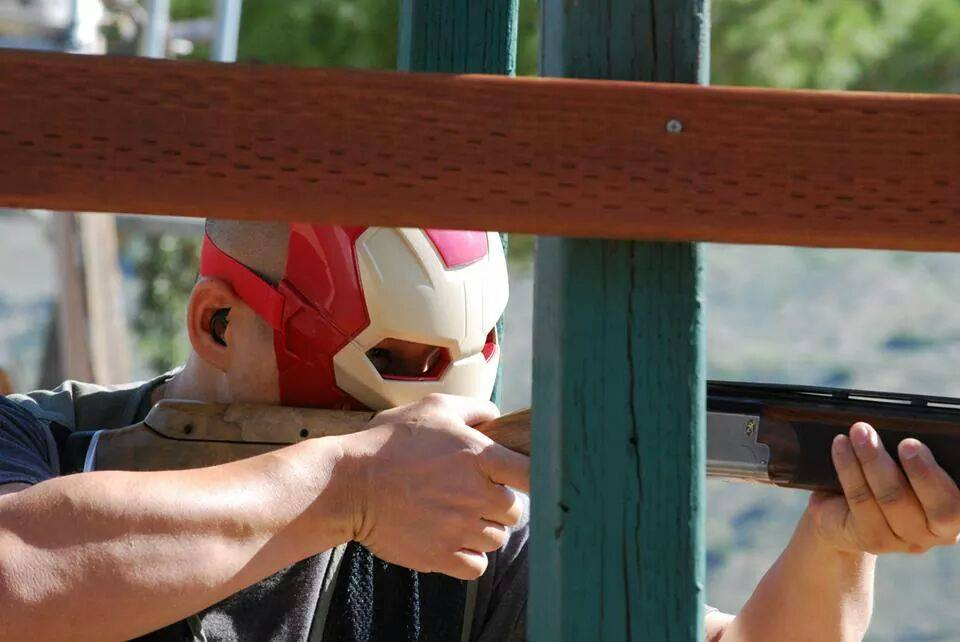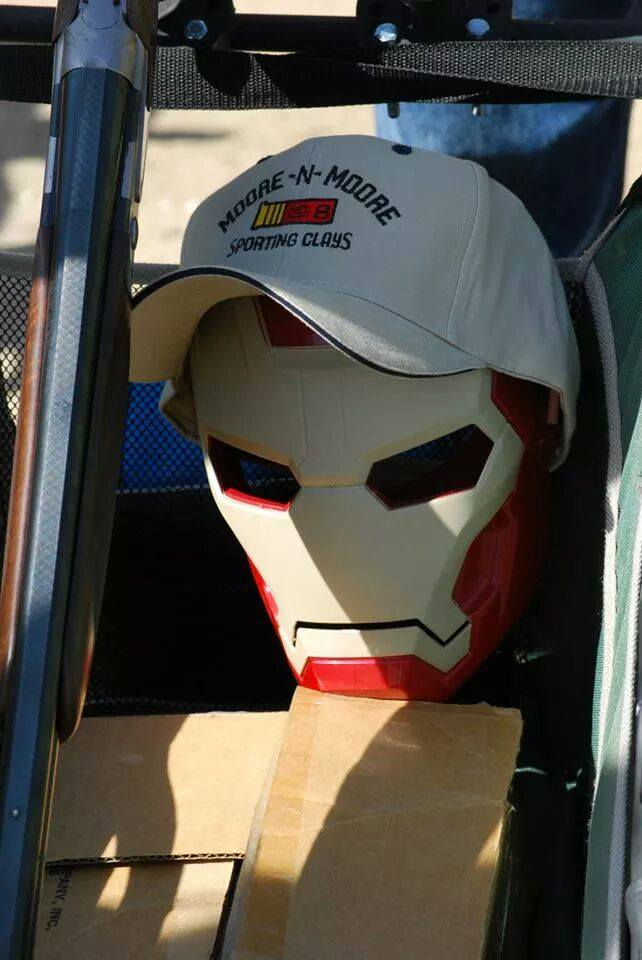From → Hacks

1.Sorry about the C#, dude 😉 Going to look at this again tomorrow when my brain can math.

•lol. C#. smh

2.Was very surprised to have have you pop up in my RSS. Great to have you back.

•Thank you aregtc. It’s great to be back

3.Eddie—very impressive data—reminds me of my old Trigonometry and Physics classes taken way too many years ago. Physics is very applicable to clays shooting. You are entirely correct—-the lead is all about the laws of Physics as the target moves through the path of the angle. Our job as shooters is to intuitively take that information and allow for the variables of wind, target arc, etc. —which you have alluded to. For reading material on this intuitive side, Dan Carlisle’s “The Secret of the Triangle” is helpful.

By the way, I am glad you have found a way to de-stress from the oft-times craziness of work-life. Keep shootin’ clays–you’re doing great!
Al Hughes

•Thank you Al. It’s always good to see you at the course.

4.very impressive, as time permits and progresses, I will figure more into this solution

5.Based on the results of my test, at 20 yards, 30 yards, 40 yards, and 50 yards, the leads were 3 ft, 4.5 ft, 6 ft, and 7.5 ft respectively. Even more interesting is that the lead angles for each of these shots were virtually the same at 2.87 degrees! To get a better understanding of how to visualize 2.87 degrees, I added a “angle_to_thumbs” conversion method which returns 1.43 thumbs. What does that mean? If you hold your arm straight out in front of you and put your thumb up, the width of your thumb is approximately 2 degrees based on this link. So imagine, 1.43 thumbs; That is your visual lead. (your thumb width may vary. Mine happens to be smaller than 2 degrees)

So far, all the calculations are correct, but there is one gaping flaw: The physics aspect is incorrect (or non-existent rather). These numbers apply if clay targets and shot didn’t decelerate and were not affected by air resistance and gravity. Unfortunately, they do. So how do we adjust these calculations to take drag into consideration?

F_D = \frac{C_D\rho A v^2}{2}

where FD is the drag force, CD is the drag coefficient, ρ is the density of air, A is the cross-sectional area of the projectile, and v is the velocity of the target. The drag coefficient is a function of things like surface roughness, speed, and spin. Even if we found an approximate drag coefficient, to further complicate things, one cannot simply plug the values into the equation and solve. Since the velocity changes at each moment (deceleration), the equation must be rewritten as a differential equation to be useful.

This is where I stop and let the reader take over the problem. Here are some good resources on drag force and drag coefficient:

6.Please check out this web site, and call the number. Dragonfiresights.com I would like to talk to you.
Thanks Cal Jones.

7.This is explained in “You’re Behind it!” The Unit Lead system for sporting clays.

8.and also http://www.Clazer.com

9.Genuinely, I’m amazed. You can’t expect to work out all of those equations everytime you go to pull the trigger..

I’ve just had a lesson with a professional instructor, 50yrs experience. His advice…

Look at it. Shoot it. Keep the gun moving.

..But until then good luck with your drag coefficient.

•Thanks Paul. I agree with your shooting advice. The best way to shoot is to not think. This post wasn’t meant to teach a shooter to perform such calculations when they shoot. Rather, it’s for those interested in the physics behind the lead as well as spread awareness of the sport and allow outsiders to appreciate its level of difficulty.

•I think my total lack of maths skill rules me out! It’s definitely got me thinking about How It all works.
Also just realised how old the thread was… Maybe my time keeping isn’t great either 🙂

10.A MUCH simpler approach is to take the ratio of the velocity of the clay target perpendicular to your line of sight to the shotgun shell velocity. (Ratio=Perp vel of clay/shell velocity). This is the lead angle in radians. To convert to degrees (if you want degrees, I don’t. I like radians) multiply this ratio by 57.29578 degrees/radian. Using your inputs I get 2.87 degrees, the same as your answer with a lot less work. Note that this result is independent of distance! Which is why your results were the same.

11.12.•